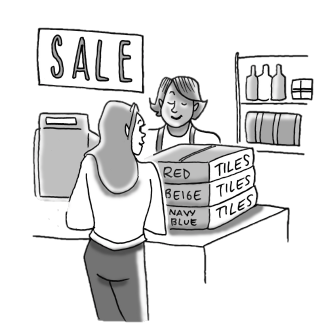### Home > ACC7 > Chapter 2 Unit 1 > Lesson CC3: 2.1.3 > Problem2-31

2-31.Write an equation and solve it to find the answer to the question below. Use the 5-D Process to help you write the equation, if needed. Remember to define your variable and to write your answer in a complete sentence.

Susan is buying three different colors of tiles for her kitchen floor. She is buying $25$ more red tiles than beige tiles, and three times as many navy-blue tiles as beige tiles. If Susan buys $435$ tiles altogether, how many tiles of each color does she buy?

Choose a number for the beige tiles.

Add $25$ to the number of beige tiles to give you the number of red tiles.

Multiply the number of beige tiles by $3$ to get the number of navy-blue tiles.

Add the beige, red, and navy-blue tiles together to get the total number of tiles Susan bought. Did your total come out to $435$? If not, try another number for the beige tiles.

Use the eTool below to input the points into the table and graph the results.
Click the link at right for the full version of the eTool: 2-31 HW eTool (Desmos)